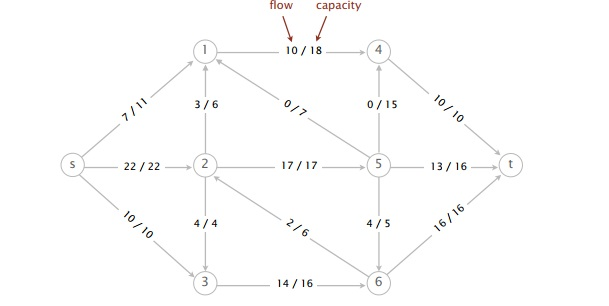### Key Concepts

Maxflow, mincut, and Ford-Fulkerson basics

• What is an st-flow f? Assignment of value to every edge.
• What is an st-cut (A, B)? Partition of vertices into two sets A and B, with s in A and t in B.
• What is the maxflow problem? What is the mincut problem?
• What is the maxflow-mincut theorem?
• How do we find an augmenting path from s to t? BFS, but with the special rule that we don't traverse a forward edge if full or a backward edge if empty.
• Given a maxflow, how can we compute a mincut? Try to find an augmenting path using algorithm above. The s side of the st-cut is every vertex reachable from the source.

Ford-Fulkerson details

• How do we know that Ford-Fulkerson terminates when all capacities are integers?
• How do we know that Ford-Fulkerson computes a maxflow (assuming it terminates)?
• What are some different strategies for picking an augmenting path? Why is DFS potentially very bad?

Residual network

• Represent spare capacity on each edge, in both forward and backward directions.
• Use mutable FlowEdge instead of immutable DirectedEdge.
• Edge v->w appears in both v's adjacency list and w's adjacency list. Why?

### Recommended Problems

#### C level

1. Consider the following st-flow network and feasible flow f.1. What is the value of the flow f?
2. Perform one iteration of the Ford-Fulkerson algorithm, starting from the flow f. Give the sequence of vertices on the augmenting path.
3. What is the value of the maximum flow?
4. List the vertices on the s side of the minimum cut.
5. What is the capacity of the minimum cut?
2. Spring 2012, #11
3. Fall 2012, #6

#### B level

1. Textbook 6.36
2. Textbook 6.38

#### A level

1. The instructors plan to grade the final, May 20. They have scheduled 8 hours for it. Each instructor prefers to grade only a certain subset of the 11 problems. They have represented their preferences in a graph in which a grader is connected to a problem if they are willing to grade it.

Each grader has a grading speed, and each problem has a grading difficulty. If a problem with difficulty d is graded by an instructor with speed s, it will take d/s minutes to grade. There are N problems and M instructors; S students took the exam.

Design an algorithm to determine if the instructors will be able to finish grading within the scheduled 8 hours, respecting their grading preferences.

What is the order-of-growth worst-case running time of your algorithm in terms of M and N? Assume that the speeds and difficulties are bounded by a constant.

Hint: formulate it as a max-flow problem and invoke a known algorithm for solving max-flow. You might want to draw a graph to illustrate how you construct a max-flow problem. Answers

2. Textbook 6.37